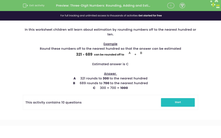# Use Rounding to Estimate Answers

In this worksheet, students will estimate the answer to an addition by rounding off numbers to the nearest hundred or ten.Key stage:  KS 2

Curriculum topic:   Number: Addition and Subtraction

Curriculum subtopic:   Estimate Calculations and Check Answers

Popular topics:   Rounding worksheets, Estimation worksheets

Difficulty level:#### Worksheet Overview

In this activity, we will be learning about estimation by rounding numbers to the nearest hundred or ten.

When rounding to the nearest ten, we look at the digit in the ones place.

If it is a 1, 2, 3,  or 4, the number rounds down to the ten below.

If the digit in the ones place is a 5, 6, 7, 8 or 9, the number rounds up to the ten above.

So, 47 rounds up to 50 because there is a 7 in the ones place, but 43 rounds down to 40 because there is a 3 in the ones place.

When rounding to the nearest hundred, we look at the digit in the tens place.

If it is a 1, 2, 3 or 4, the number rounds down to the hundred below.

If it is a  5, 6, 7, 8 or 9, the number rounds up to the hundred above.

So, 386 rounds up to 400 because there is an 8 in the tens place, but 346 rounds down to 300 because there is a 4 in the tens place.

Example

Round these numbers to the nearest hundred so that the answer can be estimated.

321 + 689   can be rounded to
 A
+
 B

A     321 rounds down to 300 to the nearest hundred

B     689 rounds up to 700 to the nearest hundred

C     300 + 700 = 1,000

Let's try some questions now!### What is EdPlace?

We're your National Curriculum aligned online education content provider helping each child succeed in English, maths and science from year 1 to GCSE. With an EdPlace account you’ll be able to track and measure progress, helping each child achieve their best. We build confidence and attainment by personalising each child’s learning at a level that suits them.

Get started